• ## 语义分割评价指标

千次阅读 2019-03-18 10:44:31
目标检测与语义分割评价指标1. 前言1.1 图像分割主要的两个方向1.2 IOU标准介绍2. 相关术语2.1 早些年2.1.1 IOU/IU2.1.2 mIoU2.1.3 AP2.1.4 AR2.1.5 Pixel Accuracy2.1.5.1 Precision2.1.5.2 Recall2.1.5.3 FP2.1....


语义分割评价指标
1. 前言1.1 图像分割主要的两个方向1.2 IOU标准介绍
2. 相关术语2.1 早些年2.1.1 IOU/IU2.1.2 mIoU2.1.3 AP2.1.4 AR2.1.5 PA2.1.5.1 Precision2.1.5.2 Recall2.1.5.3 FP2.1.5.4 FN2.1.5.5 TP2.1.5.6 TN
2.1.6 MPA2.1.7 Pixel Precision
2.2 现在2.2.1 RQ2.2.2 SQ2.2.3 PQ

参考：1 2 3 4
1. 前言
1.1 图像分割主要的两个方向
语义分割（semantic segmentation），常用来识别天空、草地、道路等没有固定形状的不可数事物（stuff）。语义分割的标记方法通常是给每个像素加上标签。实例分割（instance segmentation），人、动物或工具等可数的、独立的明显物体（things）。实例分割通常用包围盒或分割掩码标记目标。全景分割（Panoptic Segmentation）其实就是把这两个方向结合起来，生成统一的、全局的分割图像，既识别事物，也识别物体。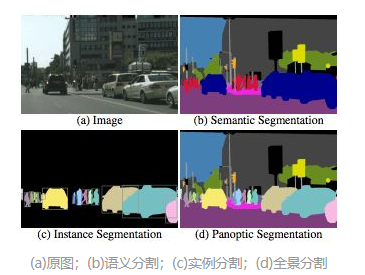1.2 IOU标准介绍
目标检测和图像分割的评价标准是一样的，核心都是使用IOU标准目标检测和图像分割使用的IOU方法都是一样的，不同的是目标检测面向判断选定框和预测框；而图像分割面向的是选定掩码和预测掩码在目标检测中，检测目标用box进行评价。我们的IOU评价方式为(将预测框和选定框放入公式中去判定)：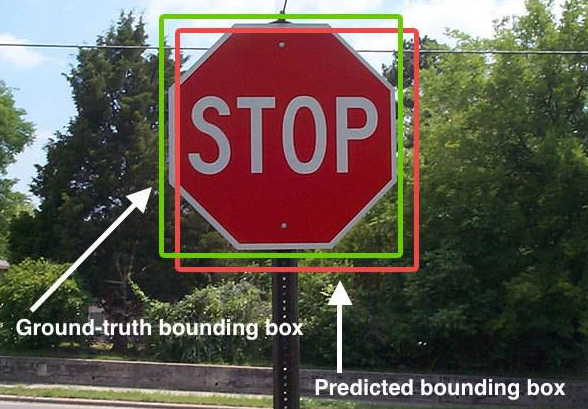而在图像分割中，我们用掩码来进行评价，IOU评价方式为(将预测掩码和选定掩码放入公式汇中去判定):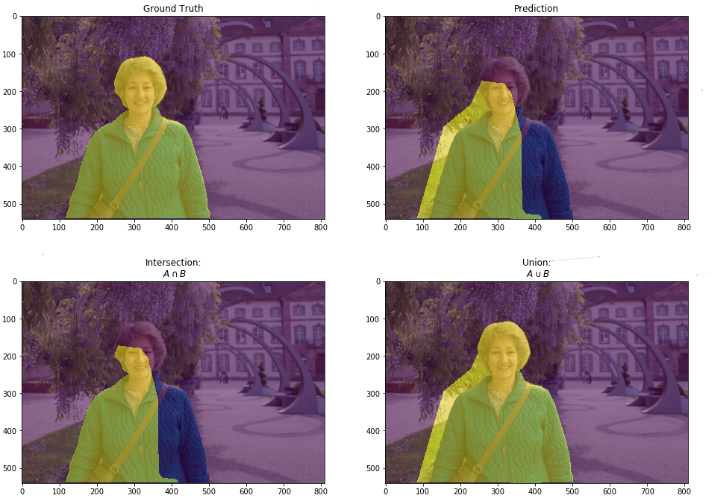2. 相关术语
2.1 早些年
2.1.1 IOU/IU
Intersection over Union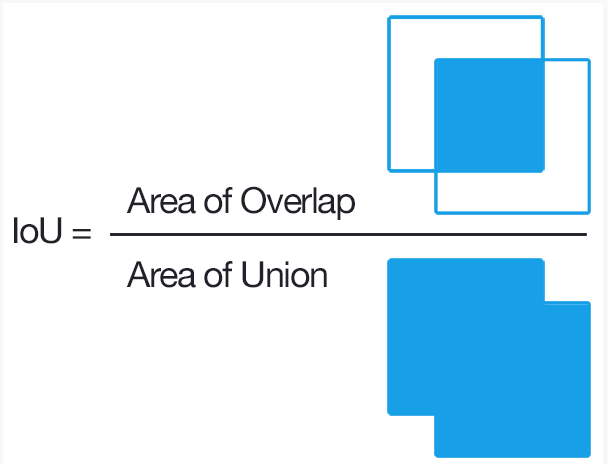IoU相当于两个区域重叠的部分除以两个区域的集合部分得出的结果， IOU算出的值score ＞ 0.5 就可以被认为一个不错的结果了用于衡量特定数据集上对象检测器的准确性
2.1.2 mIoU
mean IoU均交并比即预测区域和实际区域交集除以预测区域和实际区域的并集（计算两个集合的交集和并集之比，这俩个集合为真实值和预测值），这个比例可以变形为正真数比上真正、假负、假正（并集）之和，在每个类上计算IOU，之后平均因为我们识别或者分割图像一般都有好几个类别，所以我们把每个分类得出的分数进行平均一下就可以得到mean IoU，也就是mIoU。
2.1.3 AP
Average Precision代表平均精度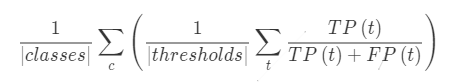2.1.4 AR
Average Recall表示平均召回率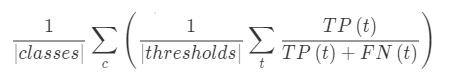2.1.5 PA
Pixel Accuracy：表示检测物体的准确度，重点判断标准为是否检测到了物体 上面所述的IoU只是用于评价一幅图的标准，如果我们要评价一套算法，并不能只从一张图片的标准中得出结论。一般对于一个数据集、或者一个模型来说。评价的标准通常来说遍历所有图像中各种类型、各种大小(size)还有标准中设定阈值.论文中得出的结论数据，就是从这些规则中得出的。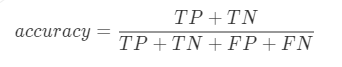2.1.5.1 Precision
准确率：表示符合要求的正确识别物体的个数占总识别出的物体个数的百分数
2.1.5.2 Recall
召回率：表示符合要求正确识别物体的个数占测试集中物体的总个数的百分数
2.1.5.3 FP
false positive误报：即预测错误(算法预测出一个不存在的物体)
2.1.5.4 FN
false negative漏报，即没有预测到(算法没有在物体规定范围内预测出该物体)
2.1.5.5 TP
true positive正确，既预测正确(算法在物体规定范围内预测出了该物体)
2.1.5.6 TN
2.1.6 MPA
mean pixel accuracy平均像素准确率MPA是对PA的改进，它是先对每个类计算PA，然后再对所有类的PA求平均
2.1.7 Pixel Precision
代表检测到所有的物体中覆盖的精确度，重点判断mask是否精确地覆盖到了该物体而像素精度则是在已经检测到的基础上(不论检测是否失误)进行评测的标准：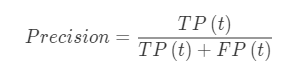上面是coco的评价标准，表示在所有检测出来的目标有多大的比率是正确的。而有些任务因为侧重不同所以公式也稍有变化。比如下面的公式，加入了FN，没有检测出来的实际物体也进行了计算
2.2 现在
2.2.1 RQ
recognition quality识别质量
2.2.2 SQ
segmentation quality分割质量
2.2.3 PQ
panoptic segmentation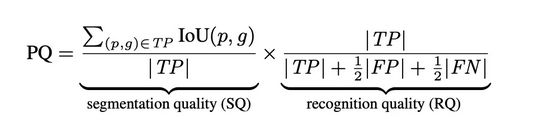展开全文• 常用来识别天空、草地、道路等没有固定形状的不可数事物（stuff）。 2、实例分割（instance segmentation）：标注方法通常是用包围盒（bbox？）或分割掩码标记目标物体；常用来识别人、动物或工具等可数的、独立的...
图像分割可分为：语义分割，实例分割，全景分割1、语义分割（semantic segmentation）：标注方法通常是给每个像素加上标签；常用来识别天空、草地、道路等没有固定形状的不可数事物（stuff）。
2、实例分割（instance segmentation）：标注方法通常是用包围盒（bbox？）或分割掩码标记目标物体；常用来识别人、动物或工具等可数的、独立的明显物体（things）。
3、全景分割（Panoptic Segmentation）：结合前面两者，生成统一的、全局的分割图像，既识别事物，也识别物体。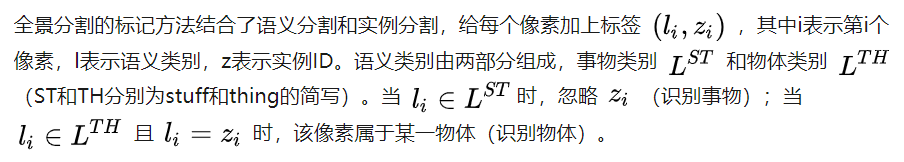图像分割评价指标：
1、IoU:  交并比，两个区域重叠的部分除以两个区域的集合部分， IOU算出的值score ＞ 0.5 就可以被认为一个不错的结果了
2、mIoU（mean IoU）：均交并比，识别或者分割图像一般都有好几个类别，把每个分类得出的分数进行平均一下就可以得到mean IoU，也就是mIoU。
3、Precision：精确率，混淆矩阵计算得出，P = TP/（TP+FP）
4、Recall：召回率，R = TP/（TP+FN）
5、Accuracy：准确率，accuracy = （TP+TN）/（TP+TN+FP+FN）
即PA（Pixel Accuracy，像素精度？标记正确的像素占总像素的比例）：表示检测物体的准确度，重点判断标准为是否检测到了物体 IoU只是用于评价一幅图的标准，如果我们要评价一套算法，并不能只从一张图片的标准中得出结论。一般对于一个数据集、或者一个模型来说。评价的标准通常来说遍历所有图像中各种类型、各种大小(size)还有标准中设定阈值.论文中得出的结论数据，就是从这些规则中得出的。
6、AP（Average Precision）：平均精度，每个类别的精确率求平均7、AR（Average Recall）：平均召回率，每个类别的召回率求平均8、MPA（mean pixel accuracy）：平均像素准确率，MPA是对PA的改进，它是先对每个类计算PA，然后再对所有类的PA求平均。10、RQ（recognition quality）识别质量
SQ（segmentation quality）分割质量
PQ（Panoptic Quality）全景质量：进一步评估分割和识别环节的表现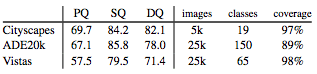人类全景分割的表现（PQ,SQ,RQ）
IoU(p,g)是预测的分割p和GroundTruth g的交并比, TP是指IoU>0.5的分割结果，FP (False Positives)，和FN (False Negatives)如下图所示：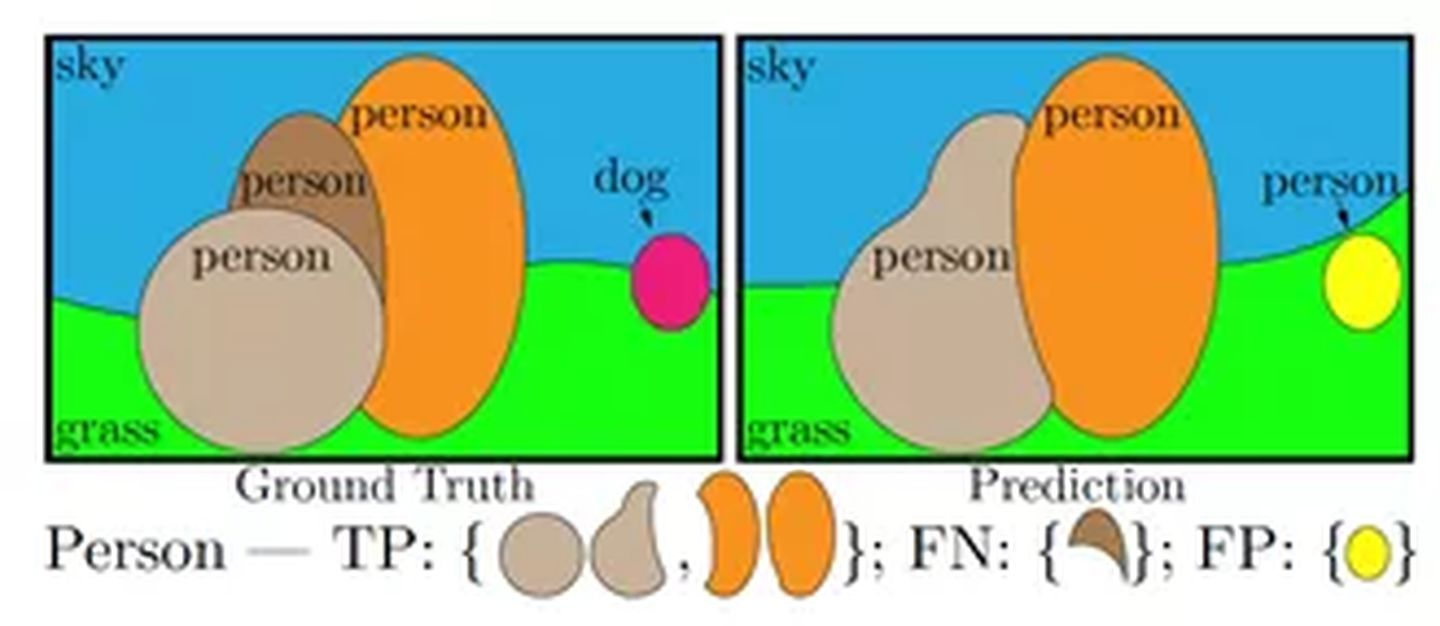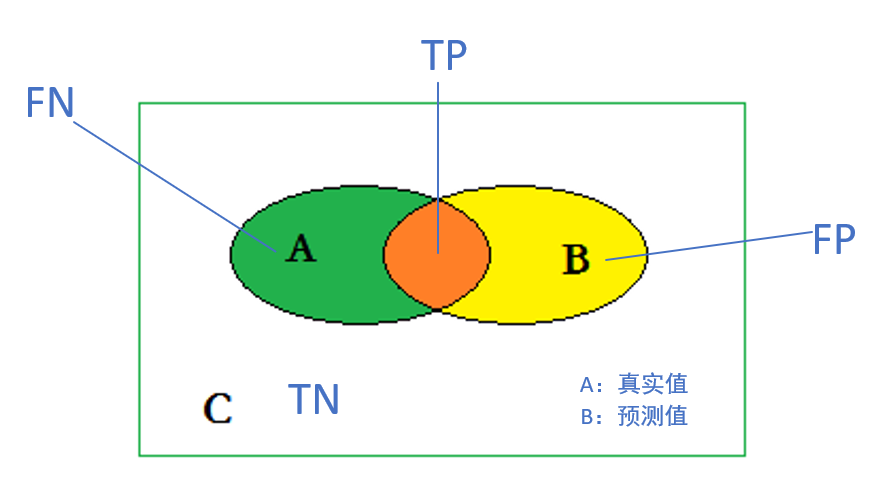评价分割系统的标准：执行时间（硬件的大致描述），内存占用（极值和均值），精确度（如下）
Pixel Accuracy(PA，像素精度)：这是最简单的度量，为标记正确的像素占总像素的比例。 Mean Pixel Accuracy(MPA，均像素精度)：是PA的一种简单提升，计算每个类内被正确分类像素数的比例，之后求所有类的平均。  Mean Intersection over Union(MIoU，均交并比)：为语义分割的标准度量。其计算两个集合的交集和并集之比，在语义分割的问题中，这两个集合为真实值（ground truth）和预测值（predicted segmentation）。这个比例可以变形为正真数（intersection）比上真正、假负、假正（并集）之和。在每个类上计算IoU，之后平均。     Frequency Weighted Intersection over Union(FWIoU，频权交并比):为MIoU的一种提升，这种方法根据每个类出现的频率为其设置权重。   在以上所有的度量标准中，MIoU由于其简洁、代表性强而成为最常用的度量标准，大多数研究人员都使用该标准报告其结果。

参考文章：https://blog.csdn.net/qq_40234695/article/details/88633094
https://blog.csdn.net/qq_37274615/article/details/78957962
https://www.zhihu.com/question/265189982/answer/462858401
展开全文• ## 图像分割性能评价

万次阅读 2015-07-09 20:45:11
采用定量的方式计算分割结果图像的性能指标从而评价分割的效果，具有客观、重复等优点。
采用

定量
$\huge{定量}$的方式计算分割结果图像的性能指标，并以此评价分割的效果，具有客观、可重复等优点。
根据是否需要理想分割的参考结果图像，可将评价方法分为两类：
无监督评价法。通过分割结果图像的

质量参数
$\huge{质量参数}$来评价相应的分割算法。有监督评价法。将算法分割结果图像

与理想分割
$\huge{与理想分割}$的参考图像进行

对比
$\huge{对比}$。
1. 无监督评价法
无监督评价法通过直接计算分割结果图像的特征参数来评价分割效果，其优势在于不需要理想分割的参考图像。分割结果图像的特征参数又称为指标或者测度。
无监督评价的指标一般分为：
区域内一致性指标区域间差异性指标语义性指标

以下分别介绍：

1.1 区域内一致性指标

好的分割，其分割的区域内部的特征具有均匀性和一致性。区域内一致性指标主要基于图像的灰度、颜色、纹理、熵等信息。

1.1.1 使用最大对比度评价一致性

可通过计算最大对比度评价一个区域的均匀性。  对于一副图像

I
$I$，假设分割后的二值图中有R1,R2,⋯,RM$R_1,R_2, \cdots, R_M$共

M
$M$个区域，则第k$k$个区域

Rk
$R_k$的一致性

zebk
$z_{ebk}$可以表示为：

zebk=1Nk∑i∈Rkj∈W(i)⋂Rkmax(fi−fj)

z_{ebk}=\frac{1}{N_k}\sum_{\substack{i \in R_k \\ j \in W \left(i \right) \bigcap R_k}}\max \left( f_i - f_j \right)

其中，

Nk
$N_k$为区域

Rk
$R_k$的像素总数，

i
$i$为Rk$R_k$中的像素，

fi
$f_i$为像素

i
$i$的灰度值，W(i)$W\left(i\right)$为像素

i
$i$的邻域, j$j$为像素

i
$i$包含在Rk$R_k$中的邻域像素。
分割后图像的一致性评价标准可以用各个区域

zebk
$z_{ebk}$的加权平均来表示：

Zeb=1N∑k=1MNkzebk

Z_{eb}=\frac{1}{N} \sum_{k=1}^{M} N_k z_{ebk}

其中

N
$N$为图像I$I$的像素总数。显然，对于一副分割结果图像，

Zeb
$Z_{eb}$值越小，区域内一致性越好。

1.1.2 使用方差评价一致性

区域内一致性与该区域的方差是反比关系。零方差意味着特征区域内所有像素的灰度值或其他像素特征（颜色、纹理等）相同。相反，方差值很大，则特征区域的一致性很差。
对于一个具有相同特性的区域

Rk
$R_k$，每一个像素

i
$i$对应的特征值记为fi$f_i$，则：
区域

Rk
$R_k$的平均特征值

fi¯
$\bar{f_i}$为：

fk¯=1Nk∑i∈Rkfi

\bar{f_k}=\frac{1}{N_k} \sum_{i\in R_k}f_i

Nk
$N_k$是区域

Rk
$R_k$的像素总和。

区域

Rk
$R_k$的方差

σ2k
$\sigma_k^2$为：

σ2k=1Nk∑i∈Rk(fi−fk¯)2

\sigma_k^2=\frac{1}{N_k}\sum_{i\in R_k} \left( f_i - \bar{f_k} \right)^2

评价一致性的指标定义为：

UI=1−⎛⎝∑Rk∈Iwkσ2k/E⎞⎠

U_I=1-\left( \sum_{R_k \in I } w_k \sigma_k^2 \big/ E \right)

式中，

wk
$w_k$为权重，

E
$E$为归一化因数：
E=⎛⎝∑Rk∈Iwk⎞⎠⋅(maxi∈Rkfi−mini∈Rkfi)22
E=\left(\sum_{R_k \in I}w_k \right) \cdot
\frac{\left(\displaystyle\max_{i \in R_k}f_i - \min_{i \in R_k}f_i \right)^2}
{2}

当使用

Rk
$R_k$的像素总数代替权值，即

wk=Nk
$w_k=N_k$ 时，有：

UI=1−2N∑Rk∈I∑i∈Rk⎛⎝fi−1Nk∑i∈Rkfi⎞⎠2(maxi∈Rkfi−mini∈Rkfi)2

U_I=1-\frac{2}{N}\sum_{R_k \in I}
\frac{\displaystyle \sum_{i \in R_k}\left( f_i - \frac{1}{N_k} \sum_{i \in R_k}f_i \right)^2}
{\left( \displaystyle \max_{i \in R_k}f_i - \min_{i \in R_k}f_i \right)^2}

对于一个分割结果图像，

UI
$U_I$越大，区域内一致性越好。

1.2 区域间差异性指标

对于好的分割，其分割的相邻区域间的特性具有显著的差异。区域间差异性指标主要基于灰度、颜色、重心距离等信息。对于具有

M
$M$个区域的图像I$I$，可以通过计算两区域间的不一致性获得区域间的差异性，如：

DIR=1C2M∑i=1M−1∑j=i+1M∣∣f(Ri)−f(Rj)∣∣max(x,y)∈I(g(x,y))−mini∈I(g(x,y))

DIR = \frac{1}{C_M^2}
\frac
{\displaystyle\sum_{i=1}^{M-1}\sum_{j=i+1}^M \left| f\left( R_i\right)  - f\left( R_j\right)\right|}
{\displaystyle\max_{\left( x, y\right) \in I} \left( g \left(x, y \right) \right) - \min_{i \in I} \left( g \left(x, y \right) \right)}

其中，

C2M
$C_M^2$为区域的组合数，

(x,y)
$\left( x, y \right)$是像素点坐标，

g(x,y)
$g\left( x, y \right)$是灰度特征函数，

f(Ri)
$f \left( R_i \right)$为区域特征函数，一般为区域平均灰度。

1.3 语义指标

语义指标主要基于分割目标的形状和边界平滑度等信息。  比如，定义目标的紧凑度和圆度指标：

紧凑度圆度=p2S=4πSp2

\begin{aligned}
紧凑度&=\frac{p^2}{S} \\
圆度&=\frac{4\pi S}{p^2}
\end{aligned}

其中，

S
$S$为分割目标的面积，p$p$为该目标的周长。

2. 有监督评价法

有监督评价的指标主要基于算法分割图像与参考图像两者的相似度或差异度，相似度越大或差异度越小，分割算法越好。

参考资料:

谢凤英. 数字图像处理与应用. 电子工业出版社, 2014.
展开全文图像处理
• 图像分割评价标准 。具体代码见链接： https://pan.baidu.com/s/1C7BWIebYbE4EJIoGKtjLSA ，密码是：gjlb，内容如下： （一）Matlab 具体的代码实现如下： 主函数demo： clc;clear all;close all; %% step ...
转载自GeekZW

                **转载自  https://blog.csdn.net/zichen_ziqi/article/details/80408465**

机器学习&图像分割——模型评价总结（含完整代码）

模型评价的方法指标有很多，如：PR-curve，MAE，ROC，Precision，Recall，AUC，AP，mAP，DSI，VOE，RVD等等；本文旨在介绍机器学习&图像分割的模型评价指标，包括完整代码，持续更新中......码字不易，若有帮助，恳请点赞，?????????

问题1：在显著性检测方面，假设现有三种方法（DSR，GMA，MCA）检测标准数据库中的部分图像（还有原图与真值图），分别得到三种结果，如何求三种方法与真值图之间的MAE（平均绝对误差），并绘制PR-curve曲线图呢？
（1）image原图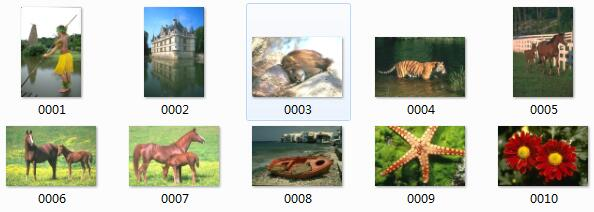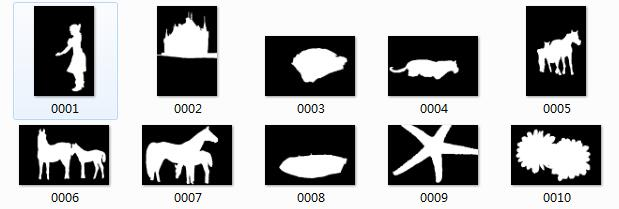（3）resultsDSR图像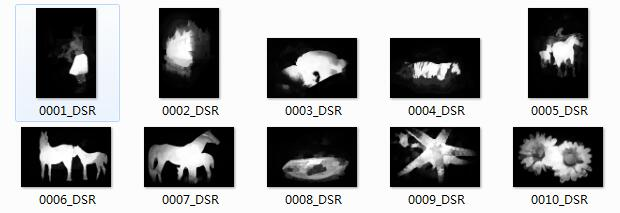（4）resultsGMA图像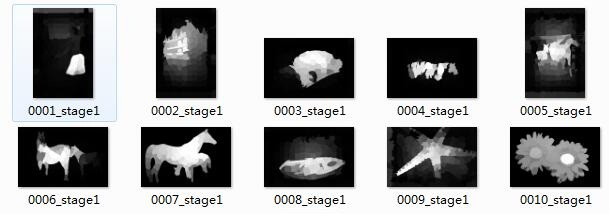（5）resultsMCA图像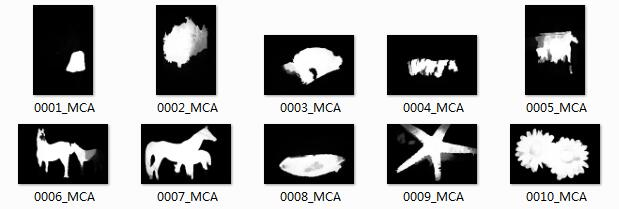文件夹内容如下图，链接：https://pan.baidu.com/s/1Wg_necXBCYsCk7pf2s7gog 或PR-curve绘制，密码：lka7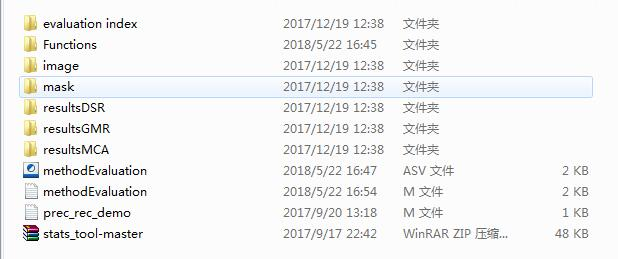输出结果为：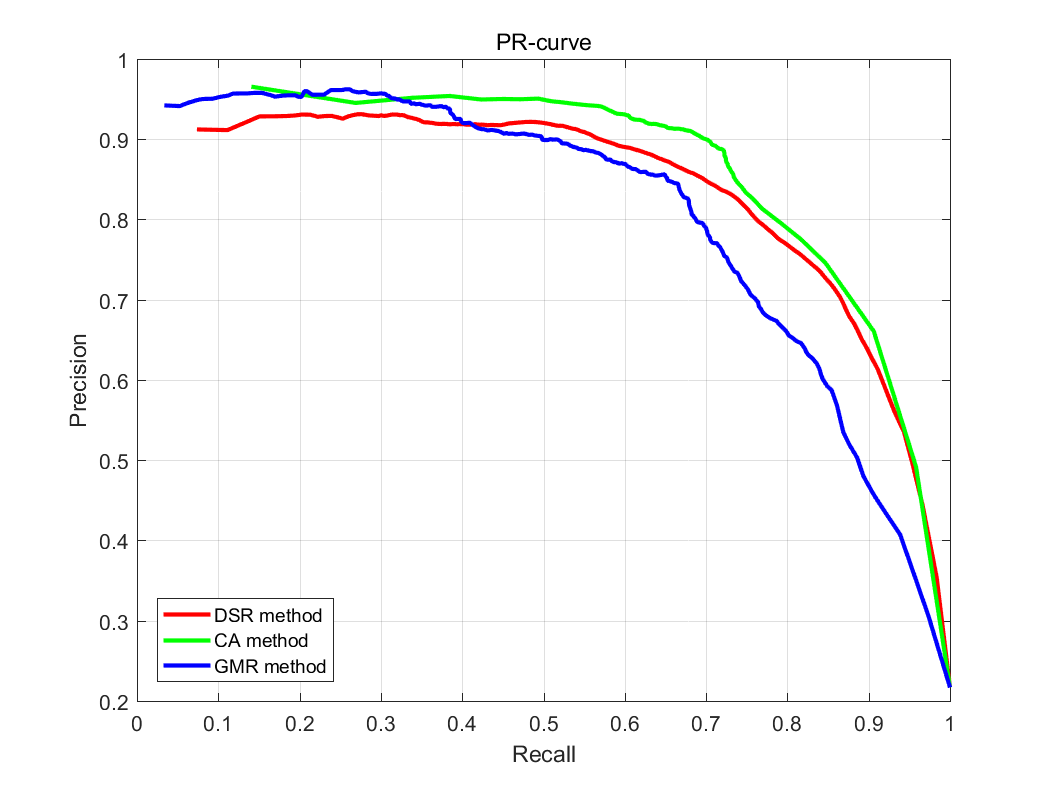具体实现——Matlab代码如下：

clear all;clc;close all;

'Functions\');%加载文件夹Functions中的函数

%% 三种方法得到的结果路径，以及真值图路径

result1 =
'resultsDSR\';

result2 =
'resultsMCA\';

result3 =
'resultsGMR\';

%% 创建文件夹evaluation index，目的是保存PR曲线图

newFolder =
'evaluation index';

if ~exist(newFolder)

mkdir(newFolder);

end

%% Evaluation index 1: evaluating MAE

resultSuffixDSR =
'_DSR.png';

resultSuffixMCA =
'_MCA.png';

resultSuffixGMR =
'_stage1.png';

gtSuffix =
'.png';

maeDSR = CalMeanMAE(result1, resultSuffixDSR, mask, gtSuffix);

maeMCA = CalMeanMAE(result2, resultSuffixMCA, mask, gtSuffix);

maeGMR = CalMeanMAE(result3, resultSuffixGMR, mask, gtSuffix);

%% Evaluation index 2: ploting PR curve

[rec1, prec1] = DrawPRCurve(result1, resultSuffixDSR, mask, gtSuffix,
true,
true,
'r');

hold on

[rec2, prec2] = DrawPRCurve(result2, resultSuffixMCA, mask, gtSuffix,
true,
true,
'g');

hold on

[rec3, prec3] = DrawPRCurve(result3, resultSuffixGMR, mask, gtSuffix,
true,
true,
'b');

hold off;

grid on;

box on;

xlabel(
'Recall');

ylabel(
'Precision');

% title(strcat(
'PR-curve',
'  ( ',sprintf(
' MAE = %1.6f ',maeDSR),
' )'));

title(
'PR-curve');

lg = legend({
'DSR method',
'CA method',
'GMR method'});

set(lg,
'location',
'southwest');

k=1.2;

set(gcf,
'units',get(gcf,
'paperunits'));

set(gcf,
'paperposition',get(gcf,
'position')*k);

saveas(gcf,strcat(newFolder,
'\PR-curve',
'.bmp'));

问题2：医学图像分割领域中的模型评价指标总结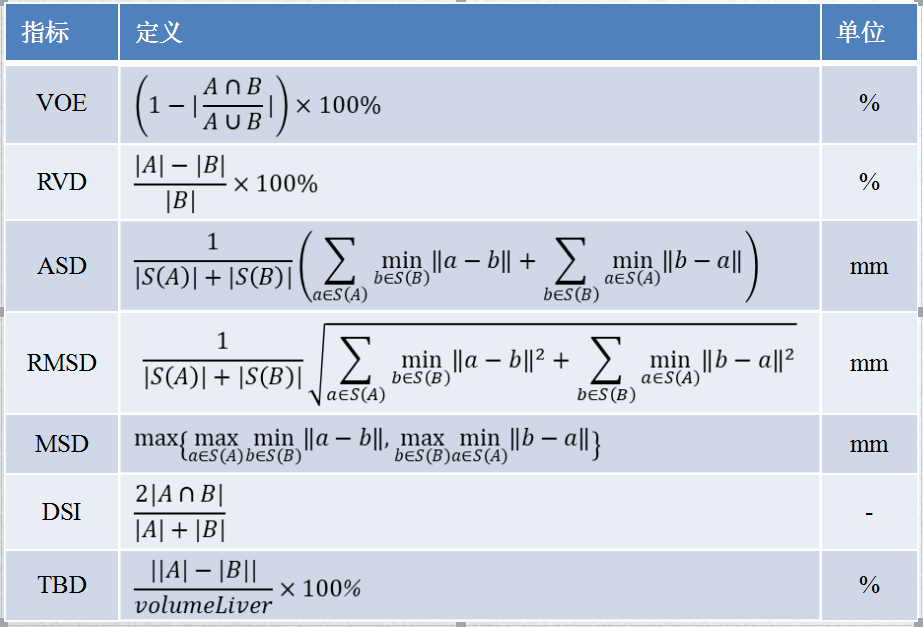强烈建议参照以下两篇文章：
《Performance measure characterization for evaluating neuroimage segmentation algorithms》
《Metrics for evaluating 3D medical imagesegmentation: analysis, selection, and tool》
度量指标分析工具github:https://github.com/Visceral-Project/EvaluateSegmentation
1、医学图像比赛（ISBI2017或者MICCAI2007）中常用到的几个度量指标：DICE，VOE，RVD，ASD，MSD等等；如何编程实现？（Matlab代码与Python代码）
符号定义：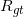：  代表 ground truth的分割结果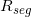：代表预测的分割结果
（1）DICE（值域为[0,1]）: 使用频率最高。数学定义如下，具体表示两个物体相交的面积占总面积的比值，完美分割该值为1。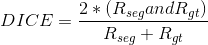（2）VOE（volumetric overlap error）： 与DICE类似，数学定义如下，它将and操作换成了减法操作，以此来代表错误率。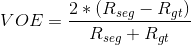（3）RVD（relative volume difference）：表示两者体积之间的差异，数学定义如下。（4）ASD（average symmetric surface distance）： 先定义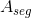代表的是预测的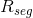中的边界的像素，同样地可以得到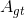的定义。然后对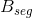的定义，同理可得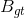的定义。那么ASD的数学定义为：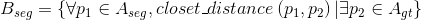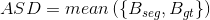（5）MSD（maximum symmetric surface distance）：与ASD定义比较类似，只不过把计算平均的操作替换成了计算最大值的操作。其数学定义为：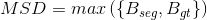参照博客：图像分割评价标准。具体代码见链接：https://pan.baidu.com/s/1C7BWIebYbE4EJIoGKtjLSA，密码是：gjlb，内容如下：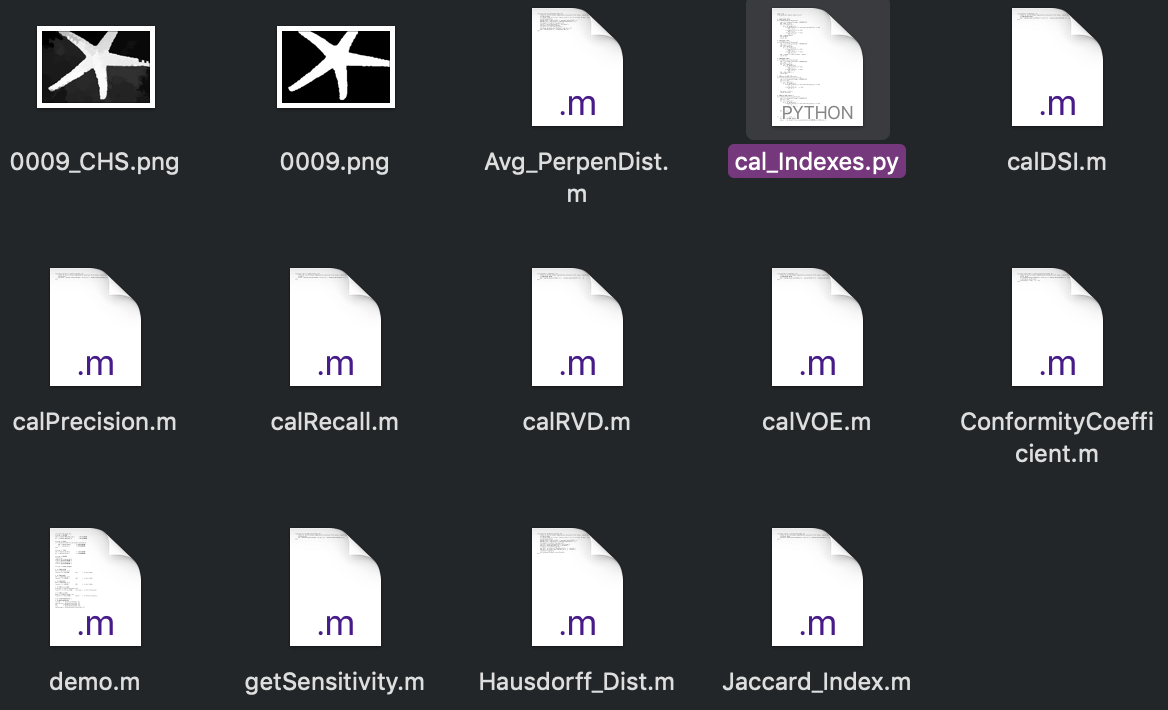（一）Matlab 具体的代码实现如下：
主函数demo：

clc;clear all;close all;

%%
step
1：读入图像

'0009_CHS.png');      % 读入分割图像

'0009.png');          % 读入真值图像

%%
step
2：灰度化

if (length(size(SEG))>
2 && length(size(GT))>
2)

SEG = im2gray(SEG);            % 灰度化分割图像

GT  = im2gray(GT);             % 灰度化真值图像

end

%%
step
3：二值化

SEG = imbinarize(SEG);             % 二值化分割图像

GT  = imbinarize(GT);              % 二值化真值图像

%%
step
4：画图预览

figure(
1),

subplot(
121),imshow(SEG);

title(
'二值化后的分割图像');

subplot(
122),imshow(GT);

title(
'二值化后的真值图像');

%%
step
5：计算各个评价指标

% (
1)计算DICE系数

DSI = calDSI(SEG, GT);

fprintf(
'(1) DICE系数：        DSI       =  %.4f\n',DSI);

% (
2)计算VOE系数

VOE = calVOE(SEG, GT);

fprintf(
'(2) VOE系数：         VOE       =  %.4f\n',VOE);

% (
3)计算RVD系数

RVD = calRVD(SEG, GT);

fprintf(
'(3) RVD系数：         RVD       =  %.4f\n',RVD);

% (
4)计算Precision系数

Precision = calPrecision(SEG, GT);

fprintf(
'(4) Precision系数：   Precision =  %.4f\n',Precision);

%（
5）计算Recall系数

Recall = calRecall(SEG, GT);

fprintf(
'(5) Recall系数：      Recall    =  %.4f\n\n\n',Recall);

disp(
'其他评价指标的计算');

% (
6)其他评价指标的计算

jaccard     = Jaccard_Index(SEG, GT)

sensitivity = getSensitivity(SEG, GT)

hd          = Hausdorff_Dist(SEG, GT)

apd         = Avg_PerpenDist(SEG, GT)

confm_index = ConformityCoefficient(SEG, GT)

先看运行结果：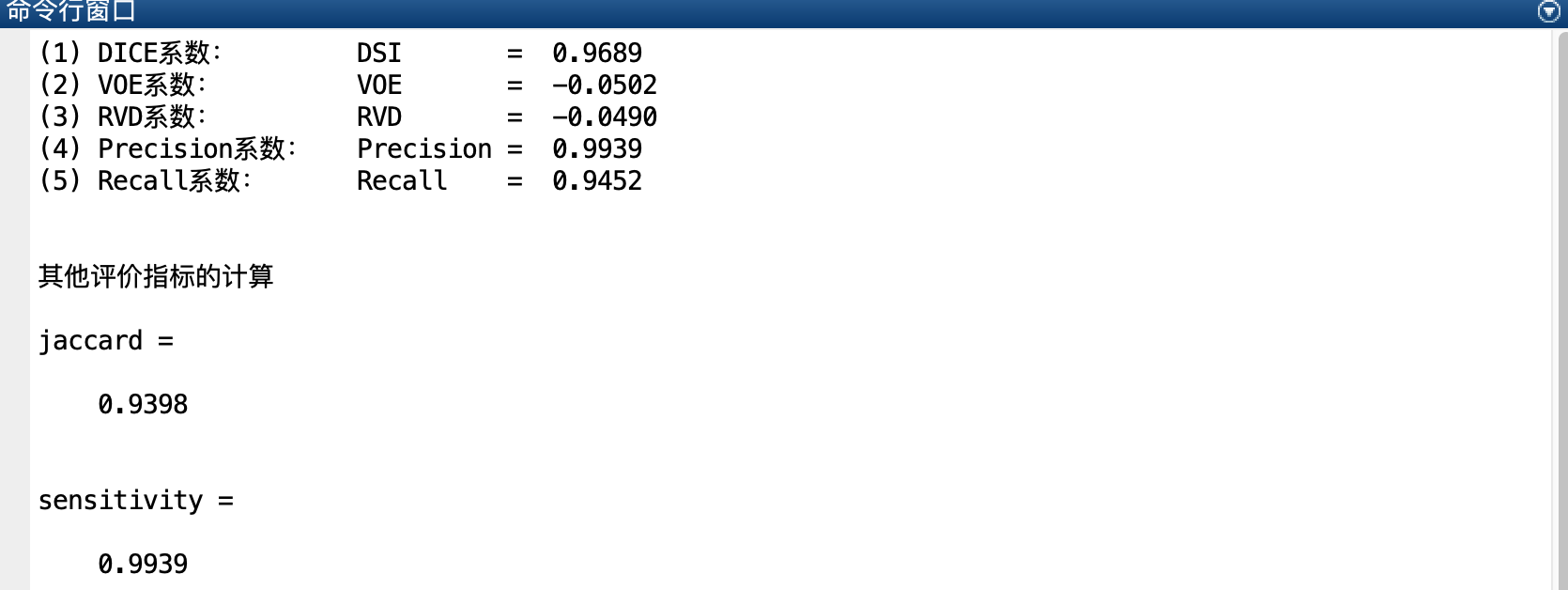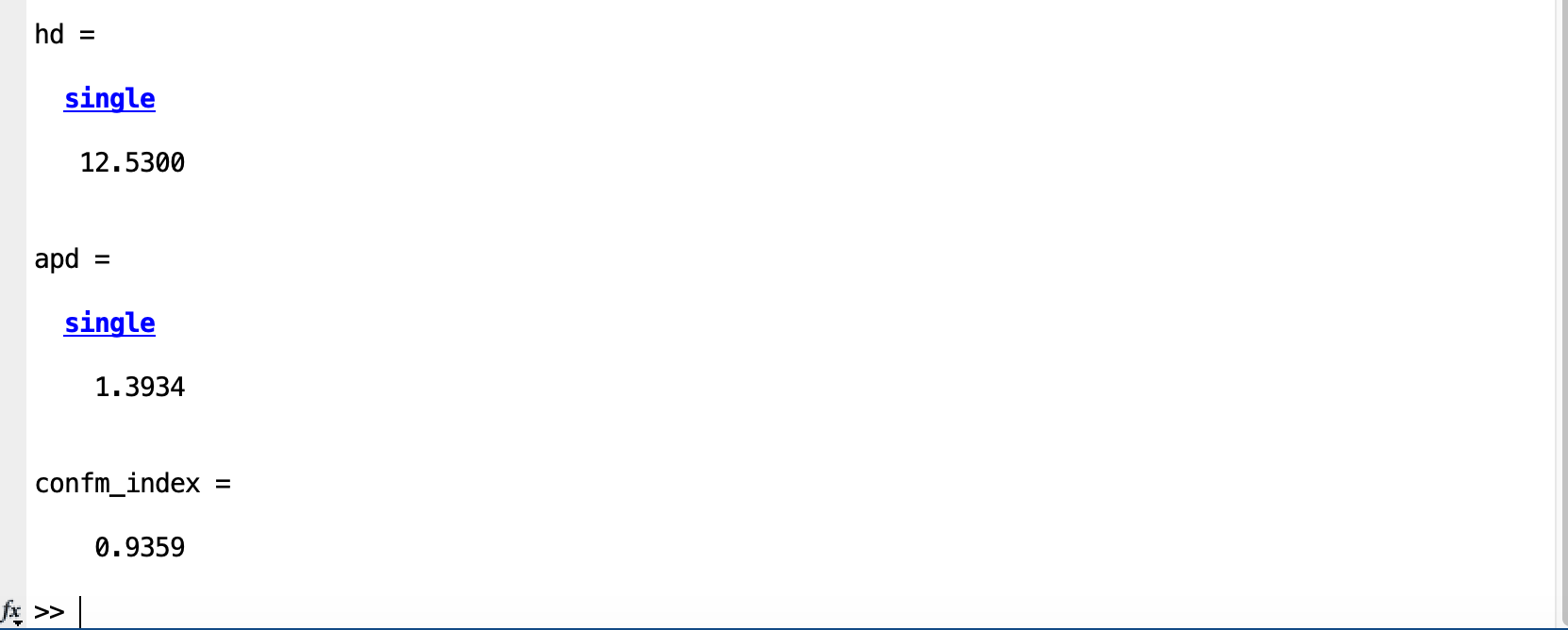其中调用函数文件分别为：
（a）calDSI函数文件：

function DSI = calDSI(SEG, GT)

% SEG, GT are the binary segmentation and ground truth areas, respectively.

% 计算DICE系数，即DSI

DSI = 2*double(sum(uint8(SEG(:) & GT(:)))) / double(sum(uint8(SEG(:))) + sum(uint8(GT(:))));

end

(b) calVOE函数文件：

function VOE = calVOE(SEG, GT)

% SEG, GT are the binary segmentation and ground truth areas, respectively.

% 计算VOE系数，即VOE

VOE = 2*double(sum(uint8(SEG(:))) - sum(uint8(GT(:)))) / double(sum(uint8(SEG(:))) + sum(uint8(GT(:))));

end

(c) calRVD函数文件：

function RVD = calRVD(SEG, GT)

% SEG, GT are the binary segmentation and ground truth areas, respectively.

% 计算RVD系数，即RVD

RVD = double(sum(uint8(SEG(:))) ) / double(sum(uint8(GT(:)))) - 1;

end

(d) calPrecision函数文件：

function precision = calPrecision(SEG, GT)

% SEG, GT are the binary segmentation and ground truth areas, respectively.

% precision

precision = double(sum(uint8(SEG(:) & GT(:)))) / double(sum(uint8(SEG(:))));

end

(e) calRecall函数文件：

function recall = calRecall(SEG, GT)

% SEG, GT are the binary segmentation and ground truth areas, respectively.

% recall

recall = double(sum(uint8(SEG(:) & GT(:)))) / double(sum(uint8(GT(:))));

end

(f) 其他指标的函数文件见以上链接。
（二）Python代码如下：

import cv2

from matplotlib
import pyplot
as plt

# 计算DICE系数，即DSI

def calDSI(binary_GT,binary_R):

row, col = binary_GT.shape
# 矩阵的行与列

DSI_s,DSI_t =
0,
0

for i
in range(row):

for j
in range(col):

if binary_GT[i][j] ==
255
and binary_R[i][j] ==
255:

DSI_s +=
1

if binary_GT[i][j] ==
255:

DSI_t +=
1

if binary_R[i][j]  ==
255:

DSI_t +=
1

DSI =
2*DSI_s/DSI_t

# print(DSI)

return DSI

# 计算VOE系数，即VOE

def calVOE(binary_GT,binary_R):

row, col = binary_GT.shape
# 矩阵的行与列

VOE_s,VOE_t =
0,
0

for i
in range(row):

for j
in range(col):

if binary_GT[i][j] ==
255:

VOE_s +=
1

if binary_R[i][j]  ==
255:

VOE_t +=
1

VOE =
2*(VOE_t - VOE_s)/(VOE_t + VOE_s)

return VOE

# 计算RVD系数，即RVD

def calRVD(binary_GT,binary_R):

row, col = binary_GT.shape
# 矩阵的行与列

RVD_s,RVD_t =
0,
0

for i
in range(row):

for j
in range(col):

if binary_GT[i][j] ==
255:

RVD_s +=
1

if binary_R[i][j]  ==
255:

RVD_t +=
1

RVD = RVD_t/RVD_s -
1

return RVD

# 计算Prevision系数，即Precison

def calPrecision(binary_GT,binary_R):

row, col = binary_GT.shape
# 矩阵的行与列

P_s,P_t =
0,
0

for i
in range(row):

for j
in range(col):

if binary_GT[i][j] ==
255
and binary_R[i][j] ==
255:

P_s +=
1

if binary_R[i][j]   ==
255:

P_t +=
1

Precision = P_s/P_t

return Precision

# 计算Recall系数，即Recall

def calRecall(binary_GT,binary_R):

row, col = binary_GT.shape
# 矩阵的行与列

R_s,R_t =
0,
0

for i
in range(row):

for j
in range(col):

if binary_GT[i][j] ==
255
and binary_R[i][j] ==
255:

R_s +=
1

if binary_GT[i][j]   ==
255:

R_t +=
1

Recall = R_s/R_t

return Recall

if __name__ ==
'__main__':

# step 1：读入图像，并灰度化

'0009.png',
0)

'0009_CHS.png',
0)

# imgray = cv2.cvtColor(img,cv2.COLOR_BGR2GRAY)   # 灰度化

# img_GT = img_GT[:,:,[2, 1, 0]]

# img_R  = img_R[:,: [2, 1, 0]]

# step2：二值化

# 利用大律法,全局自适应阈值 参数0可改为任意数字但不起作用

ret_GT, binary_GT = cv2.threshold(img_GT,
0,
255, cv2.THRESH_BINARY | cv2.THRESH_OTSU)

ret_R, binary_R   = cv2.threshold(img_R,
0,
255, cv2.THRESH_BINARY | cv2.THRESH_OTSU)

# step 3： 显示二值化后的分割图像与真值图像

plt.figure()

plt.subplot(
121),plt.imshow(binary_GT),plt.title(
'真值图')

plt.axis(
'off')

plt.subplot(
122),plt.imshow(binary_R),plt.title(
'分割图')

plt.axis(
'off')

plt.show()

# step 4：计算DSI

print(
'（1）DICE计算结果，      DSI       = {0:.4}'.format(calDSI(binary_GT,binary_R)))
# 保留四位有效数字

# step 5：计算VOE

print(
'（2）VOE计算结果，       VOE       = {0:.4}'.format(calVOE(binary_GT,binary_R)))

# step 6：计算RVD

print(
'（3）RVD计算结果，       RVD       = {0:.4}'.format(calRVD(binary_GT,binary_R)))

# step 7：计算Precision

print(
'（4）Precision计算结果， Precision = {0:.4}'.format(calPrecision(binary_GT,binary_R)))

# step 8：计算Recall

print(
'（5）Recall计算结果，    Recall    = {0:.4}'.format(calRecall(binary_GT,binary_R)))

运行结果：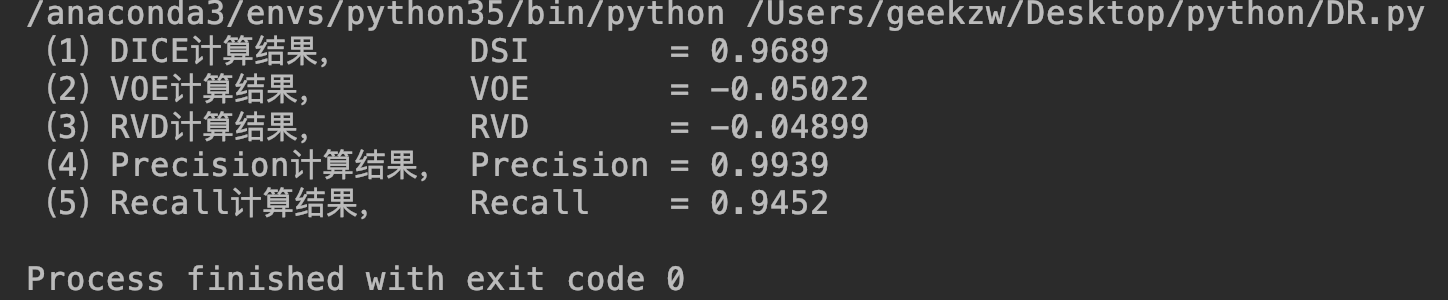2、分割精度、过分割率、欠分割率的定义
（1）分割精度：分割准确的面积占GT图像中真实面积的百分比，数学定义如下：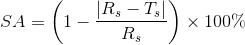其中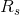表示专家手工勾画出的分割图像的参考面积，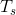表示算法分割得到的图像的真实面积，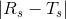表示错误分割的像素点个数。
（2）过分割率：即分割在GT图像参考面积之外的像素点的比率，数学定义如下：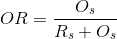其中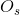表示本不应该包含在分割结果中的像素点个数，实际却在分割结果中的像素点个数。换句话讲，中的像素点出现在实际分割图像中，但不出现在理论分割图像中。
（3）欠分割率：即分割在GT图像参考面积之中欠缺的像素点的比率，数学定义如下：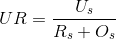其中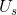表示本应该包含在分割结果中的像素点个数，实际却不在分割结果中的像素点个数。换句话讲，中的像素点出现在理论分割图像中，但不出现在实际分割图像中。

3、灵敏度与特异度的定义
假设实验图像为I，真实分割结果为G，实际分割结果为R。
（1）灵敏度：将实际是感兴趣区域的像素点正确地判断为感兴趣区域像素的比例，其衡量的是分割实验中能分割感兴趣区域的能力，其数学定义如下。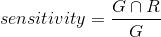（1）特异度：将实际不是感兴趣区域的像素点正确地判断为不是感兴趣区域像素的比例，其衡量的是分割实验中能正确判断不是感兴趣区域像素点的能力，其数学定义如下。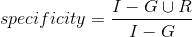Matlab的灵敏度函数：

function sen = getSensitivity(SEG, GT)

% SEG, GT are the binary segmentation and ground truth areas, respectively.

% sensitivity

sen = double(sum(uint8(SEG(:) & GT(:)))) / double(sum(uint8(SEG(:))));

end

参考网址：
1、如何在CSDN博客插入公式：http://private.codecogs.com/latex/eqneditor.php
2、图像分割结果评价：https://blog.csdn.net/zhuason/article/details/52989091
3、图像分割评价标准代码：https://blog.csdn.net/yangyangyang20092010/article/details/51637073
展开全文• 采用定量定量的方式计算分割结果图像的性能指标，并以此评价分割的效果，具有客观、重复等优点。 根据是否需要理想分割的...无监督评价法通过直接计算分割结果图像的特征参数来评价分割效果，其优势在于需要理...
• 图像分割评价指标之FWIoU图像分割评价指标之FWIoU图像分割评价指标之FWIoU
• 机器学习&图像分割——模型评价总结（含完整代码） 模型评价的方法指标有很多，如：PR-curve，MAE，ROC，Precision，Recall，AUC，AP，mAP，DSI，VOE，RVD等等； 本文旨在介绍机器学习&图像...机器学习
• 目标检测和图像分割评价标准是一样的，核心都是使用IOU标准 IOU(Intersection-Over-Union) OU即Intersection-Over-Union，IoU相当于两个区域重叠的部分除以两个区域的集合部分得出的结果 目标检测 图像分割 ...深度学习 计算机视觉
• IoU (Intersection over Union) 从字面意义上来说就是交并比，顾名思义就是两个集合的交集与两个...在语义分割任务中，通常使用预测(predict label)与真值(ground truth)之间的交并比作为评价标准，例如在PASCAL、...真负例 假正例
• 摘 要 对医学图像分割算法的客观评价是推进算法在临床上得到应用的关键。针对目前对医学图像分割方法的研究较多,而对分割算法的评价方法的研究却很少的问题,提出了一种判断和比较医学图像分割算法优劣的评价方法。...
• 从混淆矩阵出发，再看各项性能评价指标就一目了然了。 1）True positives(TP): 被正确地划分为正例的个数，即实际为正例且被分类器划分为正例的实例数（样本数）； 2）False positives(FP): 被错误地划分为正例的...目标检测 深度学习
• 图像分割评价指标---mIoU（平均交并比）计算代码（OpenCV/C++） 运行环境：Visual Studio+OpenCV+C++ 输入：准备好的Groundtruth数据+分割得到的二值图像 输出：每张分割结果的IOU值+最后输出MIOU值（平均交并比） ...OpenCV C++ mIOU 平均交并比 代码
• psnr是“Peak Signal to Noise Ratio”的缩写，即峰值信噪比，是一种评价图像的客观标准。 为了衡量经过处理后的影像品质，我们通常会参考PSNR值来衡量某个处理程序能否令人满意。PSNR的单位是dB，数值越大表示失真...PSNR SSIM
• 1. 语义分割IoU的定义 传统意义上的IoU(Intersection over Union，交并比) 直观表示: 公式: 语义分割中的IoU 在语义分割的问题中，这两个集合为真实值（ground truth）和预测值（predicted segmentation）。 ...
• 在我们使用eCognition中的多尺度分割算法分割影像时，会发现不知该如何确定各分割参数，于是有学者研究开发了ESP2工具，来帮助确定最佳分割尺度参数。本文主要对ESP2工具的使用方法及运行结果解读进行了介绍，如有...易康
• 显然合理，所以需要有一个衡量的标准。 1.分类网络： 首先要知道TP,FP,TN,FN的概念 如下图： 多种类的时候可以使用混淆矩阵来看一下分类的结果： 预测 实际 0 1 ...深度学习
• 最近在做小目标图像分割任务（医疗方向），往往一幅图像中只有一个或者两个目标，而且目标的像素比例比较小，选择合适的loss function往往可以解决这个问题。以下是我的实验比较。 场景： 1.U-Net网络 2. keras...keras dice loss focal loss
• 文章目录引言1 混淆矩阵2 语义分割PA：像素准确率CPA：类别像素准确率MPA：类别平均像素准确率IoU：交并比MIoU：平均交并比（改进，先求IoU，再求MIoU，这里有误）3 综合实例步骤一：输入真实、预测图片步骤二：求出...
• 深度学习100问Author：louwillMachine Learning Lab 语义分割作为经典的图像分割问题，其本质上还是一种图像像素分类。既然是分类，我们就...
• ## 图像分割

千次阅读 2017-08-17 16:51:40
1.数字图像分割方法概要 2.基于边界分割 2.1边缘检测 2.2边界提取（简单连接，启发式搜索，曲线拟合） 3.基于区域分割 3.1阀值分割（直方图双峰，迭代法，Ostu(大律）法，基于熵的二值方法） 3.2区域生长 ...
• 在数字图像处理中，图像分割是很关键的一步，当图像质量较好，光照很均匀的时候只需用全局阈值的方法就能很完美地完成图像分割任务，但是有些时候会遇到光照均匀的现象，这个时候就需要用一些技巧才能达...图像处理
• 本博文介绍如何手把手制作自己的数据集，并使用SegNet网络进行语义分割，如何训练，如何测试自己的分割模型。 ------------------------------------------------------------------------------------------------...
• ## 图像分割综述

万次阅读 多人点赞 2019-07-09 22:03:48
图像分割是计算机视觉研究中的一个经典难题，已经成为图像理解领域关注的一个热点，图像分割是图像分析的第一步，是计算机视觉的基础，是图像理解的重要组成部分，同时也是图像处理中最困难的问题之一。所谓图像分割...
• 利用位置信息来进行实例分割，将原图大致分为S*S个矩形框，分别对每个框进行分类和分割预测。流程如图： 输出共有两个分支，上面分支预测类别信息，输出尺寸为S * S * C：S为原图预设的分割矩形框数目；C为类别个数...算法
• 介绍SLIC超像素分割算法，给出其与openCV的接口，代码用VS2012和openCV2.4.9测试运行。opencv
• 阈值分割我们曾在3.5节学习过灰度阈值变换的相关知识， 利用灰度阈值变换分割图像就称为阈值分割， 它是一种基本的图像分割方法。 阙值分割的基本思想是确定一个阈值， 然后把每个像素点的灰度值和阈值相比较，根据...技术 图像处理
• 近年来，完整话语重写的任务引起了广泛关注。以前的方法通常将其转换为机器翻译任务，并采具有复制机制的生成模型。在本文中，作者将该任务转换为语义分割任务，引入了基于词的编辑操作，并将问题重...算法 python 计算机视觉 机器学习 人工智能...